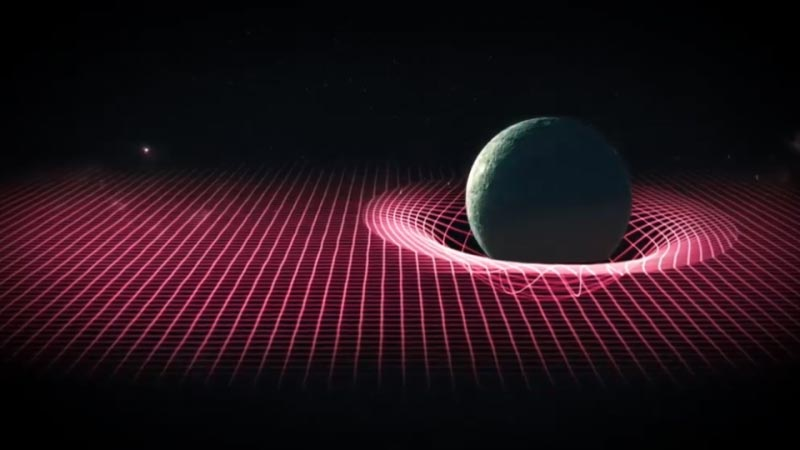# How the Universe Works: Mystery of Space-time ep.10 2018Space-time is the secret structure that controls the universe, and this strange four-dimensional substance controls time, light, and energy. It will also determine how the universe will end.

## Space-time

In physics, space-time is any mathematical model that fuses the three dimensions of space and the one dimension of time into a single four-dimensional continuum. Space-time diagrams are useful in visualizing and understanding relativistic effects such as how different observers perceive where and when events occur.

Until the turn of the 20th century, the assumption had been that the three-dimensional geometry of the universe (its description in terms of locations, shapes, distances, and directions) was distinct from time (the measurement of when events occur within the universe). However, Albert Einstein’s 1905 special theory of relativity postulated that the speed of light through empty space has one definite value, a constant, that is independent of the motion of the light source. Einstein’s equations described important consequences of this fact. The distances and times between pairs of events vary when measured in different inertial frames of reference (separate vantage points that aren’t being subjected to g‑forces but have different velocities).

Einstein’s theory was framed in terms of kinematics (the study of moving bodies), and showed how quantification of distances and times varied for measurements made in different reference frames. His theory was a breakthrough advance over Lorentz’s 1904 theory of electromagnetic phenomena and Poincaré’s electrodinamic theory. Although these theories included equations identical to those that Einstein introduced, they were essentially ad hoc models proposed to explain the results of various experiments—including the famous Michelson–Morley interferometre experiment—that were extremely difficult to fit into existing paradigms.

Tags:
Scroll to Top